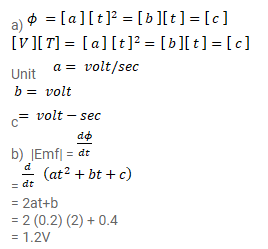# The flux of magnetic field through a closed conducting loop changes

Question:

The flux of magnetic field through a closed conducting loop changes with time according to the equation, $\phi=a t^{2}+b t+c$ a) Write the SI units of $a, b$ and $c$. b) If the magnitude of $a, b$ and $c$ are $0.20,0.40$ and $0.60$ respectively, find the induced emf at $\mathrm{t}=2 \mathrm{~s}$

Solution: